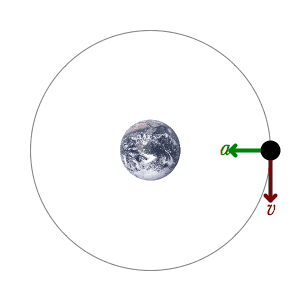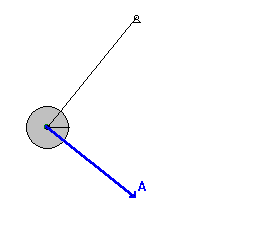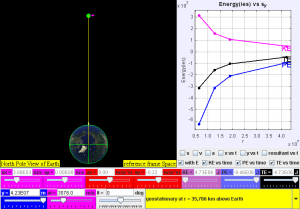# 10 Facts about Mechanical Energy

Post On: January 3, 2018
By: Andi

Facts about Mechanical Energy show you the amazing less-known information about the mixing between potential energy and kinetic energy widely recognised as mechanical energy in physical sciences. The principle of mechanical energy is that in an isolated system that is only subject to conservative forces, the mechanical energy is constant. The facts that many devices are used to convert to or from other forms of energy, for example, an electric motor converts electric energy to mechanical energy. The more know facts about mechanical energy the more inspiration you got. The presentation below talks about Mechanical Engineering.

## Facts about Mechanical Energy 1: General

Mechanical energy is sum of potential energy and kinetic energy. The principal of potential energy depends on the position of an object subjected to a conservative force, while kinetic energy widely known as the energy of motion and it depends on the speed of an object and the ability  of moving object to do working on other objects when it encounters with them.

### Facts about Mechanical Energy 2: Conservation of Mechanical Energy

The principle of conservation of mechanical energy commonly used as a fair approximation, altough energy can not be broken or made in an isolated system, yet it can be converted to another form of energy.## Facts about Mechanical Energy 3: Swinging Pendulum

Swinging pendulum often subjected to the conservative gravitational force where frictional forces like air drag and friction at the pivot are negligible, energy passes back and forth between kinetic and potential energy but never left the system. The illustratation can be seen on the picture below (in the 6th list).

### Facts about Mechanical Energy 4: Irreversibilities

In facts that the loss of mechanical energy will cause the increasing the sistem’s temperature for long term. The friction and collision between objects converted into an equal of heat. Hence, the total energy of the system remains unchange even though the mechanical energy of the system has been reduced.

## Facts about Mechanical Energy 5: Satellite

A satellite of mass at a distance from the core of the earth has kinetic energy and gravitational energy. Eventually, the mechanical energy of satellite given by suming both kinetic and potential energy.

### Facts about Mechanical Energy 6: Conversion

Yet to date, mandy technological devices convert mechanical energy into other form of energy or vice versa. For instance, a generator changes mechanical energy to electrical energy, a hydroelectric powerplant converts the mechanical enery  of water in a stored dam into electrical energy, a steam engine change the heat energy of steam into mechanical energy, and a turbine converts the kinetic energy of a stream of gas or liquid into mechanical energy, etc.## Facts about Mechanical Energy 7: Distinction from Other Types

The distinction of energy into different types often follows the boundaries of the fields of study in the natural sciences.

### Facts about Mechanical Energy 8: The Objects That Contain Mechanical Energy

All objects in motion contains mechanical energy.

## Facts about Mechanical Energy 9: The Consequence of Loses Mechanical Energy

When an object loses mechanical energy it will gain heat and increasing in temperature.